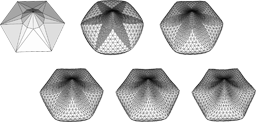# Discrete Fairing

Leif Kobbelt
Proceedings of the Seventh IMA Conference on the Mathematics of Surfaces, 1997, pp. 101-131We address the general problem of, given a triangular net of arbitrary topology in IR3 , nd a re ned net which contains the original vertices and yields an improved approximation of a smooth and fair interpolating surface. The (topological) mesh re nement is performed by uniform subdivision of the original triangles while the (geometric) position of the newly inserted vertices is determined by variational methods, i.e., by the minimization of a functional measuring a discrete approximation of bending energy. The major problem in this approach is to nd an appropriate parameterization for the re ned net's vertices such that second divided di erences (derivatives) tightly approximate intrinsic curvatures. We prove the existence of a unique optimal solution for the minimization of discrete functionals that involve squared second order derivatives. Finally, we address the e cient computation of fair nets.